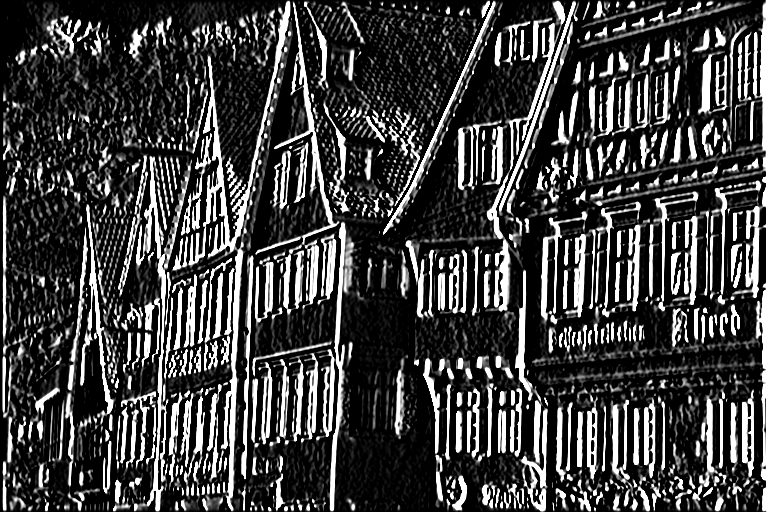## VPI - Vision Programming Interface

#### 2.3 Release

Separable Convolution

# Overview

The Separable Convolution algorithm performs a 2D convolution operation, but takes advantage of the fact that the 2D kernel is separable. The user passes one horizontal and one vertical 1D kernel. This usually leads to better performance, especially for kernels larger than 5x5. For smaller kernels, it's preferable to use Convolution algorithm with a 2D kernel directly.

Input Sobel kernel Output\begin{eqnarray*} k_{col} &=& \frac{1}{64} \begin{bmatrix} 1 \\ 6 \\ 15 \\ 20 \\ 15 \\ 6 \\ 1 \end{bmatrix} \\ k_{row} &=& \begin{bmatrix} -1 & -5 & -6 & 0 & 6 & 5 & 1 \end{bmatrix} \end{eqnarray*}# Implementation

Discrete 2D convolution is implemented using the following discrete function:

\begin{eqnarray*} I'[x,y] &=& \sum_{m=0}^{k_w} K_{row}[m] \times I[x,y-(m - \lfloor k_w/2 \rfloor)] \\ I''[x,y] &=& \sum_{m=0}^{k_h} K_{col}[m] \times I'[x-(m - \lfloor k_h/2 \rfloor),y] \end{eqnarray*}

Where:

• $$I$$ is the input image.
• $$I'$$ is the temporary image with convolution along the rows.
• $$I''$$ is the final result.
• $$K_{row}$$ is the row convolution kernel.
• $$K_{col}$$ is the column convolution kernel.
• $$k_w,k_h$$ are the kernel's width and height, respectively.
Note
Most computer vision libraries expect the kernel to be reversed before calling their convolution functions. Not so with VPI, we implement a actual convolution, not cross-correlation. Naturally, this is irrelevant if the kernel is symmetric.

# C API functions

For list of limitations, constraints and backends that implements the algorithm, consult reference documentation of the following functions:

Function Description
vpiSubmitSeparableConvolution Runs a generic 2D convolution operation over an image, optimized for separable kernels.

# Usage

Language:
1. Import VPI module
import vpi
2. Define a 3x3 separable Sobel kernel.
sobel_row = [-1, -5, -6, 0, +6, +5, +1];
sobel_col = [1/64.0, 6/64.0, 15/64.0, 20/64.0, 15/64.0, 6/64.0, 1/64.0]
3. Run separable convolution filter on input image using the CPU backend and the given kernel. Input and output are VPI images.
with vpi.Backend.CUDA:
output = input.convolution(kernel_x=sobel_row, kernel_y=sobel_col, border=vpi.Border.ZERO)
1. Initialization phase
1. Include the header that defines the needed functions and structures.
Declares functions to perform image filtering with convolution kernels.
2. Define the input image object.
VPIImage input = /*...*/;
struct VPIImageImpl * VPIImage
A handle to an image.
Definition: Types.h:256
3. Create the output image. It gets its dimensions and format from the input image.
int32_t w, h;
vpiImageGetSize(input, &w, &h);
vpiImageGetFormat(input, &type);
VPIImage output;
vpiImageCreate(w, h, type, 0, &output);
uint64_t VPIImageFormat
Pre-defined image formats.
Definition: ImageFormat.h:94
VPIStatus vpiImageGetFormat(VPIImage img, VPIImageFormat *format)
Get the image format.
VPIStatus vpiImageCreate(int32_t width, int32_t height, VPIImageFormat fmt, uint64_t flags, VPIImage *img)
Create an empty image instance with the specified flags.
VPIStatus vpiImageGetSize(VPIImage img, int32_t *width, int32_t *height)
Get the image dimensions in pixels.
4. Create the stream where the algorithm will be submitted for execution.
VPIStream stream;
vpiStreamCreate(0, &stream);
struct VPIStreamImpl * VPIStream
A handle to a stream.
Definition: Types.h:250
VPIStatus vpiStreamCreate(uint64_t flags, VPIStream *stream)
Create a stream instance.
2. Processing phase
1. Define the kernel to be used. In this case, a simple 7x7 Sobel filter.
float sobel_row = {-1, -5, -6, 0, +6, +5, +1};
float sobel_col = {1/64.f, 6/64.f, 15/64.f, 20/64.f, 15/64.f, 6/64.f, 1/64.f};
2. Submit the algorithm to the stream, passing the 1D kernels and remaining arguments. I'll be executed by the CUDA backend.
vpiSubmitSeparableConvolution(stream, VPI_BACKEND_CUDA, input, output, sobel_row, 7, sobel_col, 7, VPI_BORDER_ZERO);
VPIStatus vpiSubmitSeparableConvolution(VPIStream stream, uint64_t backend, VPIImage input, VPIImage output, const float *kernelXData, int32_t kernelXSize, const float *kernelYData, int32_t kernelYSize, VPIBorderExtension border)
Runs a generic 2D convolution operation over an image, optimized for separable kernels.
@ VPI_BACKEND_CUDA
CUDA backend.
Definition: Types.h:93
@ VPI_BORDER_ZERO
All pixels outside the image are considered to be zero.
Definition: Types.h:278
3. Optionally, wait until the processing is done.
vpiStreamSync(stream);
VPIStatus vpiStreamSync(VPIStream stream)
Blocks the calling thread until all submitted commands in this stream queue are done (queue is empty)...
3. Cleanup phase
1. Free resources held by the stream and the input and output images.
vpiImageDestroy(output);
void vpiImageDestroy(VPIImage img)
Destroy an image instance.
void vpiStreamDestroy(VPIStream stream)
Destroy a stream instance and deallocate all HW resources.

For more information, see Convolution in the "C API Reference" section of VPI - Vision Programming Interface.

# Performance

For information on how to use the performance table below, see Algorithm Performance Tables.
Before comparing measurements, consult Comparing Algorithm Elapsed Times.
For further information on how performance was benchmarked, see Performance Benchmark.

-